Definitions of Square Dance Calls and Concepts
Split Phantom Boxes Concept [C3B]

Index -->  Plus  |  A1  |  A2  |  C1  |  C2  |  C3A  |  C3B  |  C4  |  NOL  |
Definitions (Text Only) -->  Plus  |  A1  |  A2  |  C1  |  C2  |  C3A  |  C3B  |  C4  |  NOL  |
 Find call:

Split Phantom Boxes Concept -- [C3B]
(author unknown)

 \$B8@8l(B2 x 8 Matrix \$B\$+\$i(B.

2 x 8 Matrix \$B\$r(B 2 \$B\$D\$N(B 2 x 4 Matrix \$B\$KJ,\$1\$^\$9(B. Each side \$B\$N(B 2 x 4 \$B\$GF0\$-\$^\$9(B.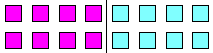Split Phantom Boxes\$B\$G\$O\$3\$l\$i\$N%]%8%7%g%s\$GF0\$-\$^\$9(B

Split Phantom Boxes In Roll Circulate: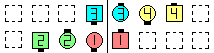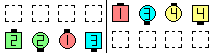Split Phantom Boxes In Roll Circulate\$B\$NA0(B \$B8e(B
Split Phantom Boxes Walk Out To A Wave: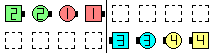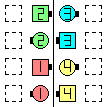Split Phantom Boxes Walk Out To A Wave\$B\$NA0(B \$B8e(B

\$BCm
• Split Phantom Boxes \$B\$O(B Split Phantom Columns| Lines | Waves [C3A] \$B\$H;w\$F\$\$\$^\$9\$,(B, \$BNY\$j9g\$o\$;\$N(B 2 x 4 formation \$B\$,(B center-to-center \$B\$G\$J\$/(B end-to-end \$B\$H\$J\$C\$F\$\$\$kE@\$,0c\$\$\$^\$9(B.
• Split Phantom Columns | Lines | Waves \$B\$HF1\$8\$h\$&\$K(B, Split Phantom Boxes \$B\$G%3!<%k\$r9T\$&\$H\$-(B, \$B<+J,\$N(B set \$B\$NH>J,\$NJ}\$KN1\$^\$kI,MW\$,\$"\$j\$^\$9(B.
• Set \$B\$rH>J,\$E\$D\$KJ,\$1\$kN{4\$\$NJI(B (\$BF|K\\$G\$O!V;0ES\$N@n!W\$H\$b8@\$o\$l\$^\$9(B) \$B\$,\$"\$k\$H9M\$(\$F\$_\$F\$/\$@\$5\$\$(B. \$B\$=\$&\$9\$l\$P(B, set \$B\$N\$b\$&(B 1 \$B\$D\$NH>J,\$NJ}\$KF~\$C\$F\$7\$^\$&\$3\$H\$rKI\$0\$3\$H\$,\$G\$-\$^\$9(B.
• \$BNr;KE*\$K(B, Split Phantom Boxes \$B\$O(B, Split Phantom Twin Boxes \$B\$H(B Divided Phantom Columns | Lines | Waves \$B\$H\$7\$FCN\$i\$l\$F\$\$\$^\$7\$?(B.

• Split Phantom formations CONCEPT [C4]: 16 \$B\$N(B spot \$B\$+\$i\$J\$k(B Phantom Formation \$B\$+\$i(B. 16-spot formation \$B\$r(B 4 spot \$B\$E\$D\$N(B 4 \$B\$D\$N(B sub-formation \$B\$XJ,\$1\$^\$9(B. \$B3F!9\$N(B sub-formation \$B\$N2s\$j\$K2>A[\$N1_\$rIA\$\$\$F\$_\$^\$7\$g\$&(B. \$B\$3\$l\$i\$N1_\$O(B, \$B\$*8_\$\$\$K8r:9\$7\$^\$;\$s(B. \$B\$^\$?(B, A - B - C - D \$B\$N3F!9\$N\$r(B 4 spot \$B\$+\$i\$J\$k%0%k!<%W\$H\$7\$F(B, ABCD \$B\$N\$h\$&\$KJB\$Y\$?\$H\$-(B, 4 \$B\$D\$N1_\$NJB\$S\$O(B 1 \$B\$D\$N??\$C\$9\$0\$J@~\$N>e\$K\$"\$j\$^\$9(B.

• Split Phantom formations \$B\$G\$O(B, 2 \$B\$D\$NNY\$j9g\$C\$?%0%k!<%W(B (\$B\$9\$J\$o\$A(B, AB and CD) \$B\$,(B, \$B<+J,\$N(B 8-spot formation \$B\$G0l=o\$KF0\$-\$^\$9(B.
• Interlocked Phantom formations \$B\$G\$O(B, once-removed \$B\$N%0%k!<%W(B (\$B\$9\$J\$o\$A(B AC and BD) \$B\$,(B, \$B<+J,\$N(B 8-spot formation \$B\$G0l=o\$KF0\$-\$^\$9(B.
• Phantom formations \$B\$G\$O(B, concentric \$B\$N%0%k!<%W(B (\$B\$9\$J\$o\$A(B, AD and BC) \$B\$,(B, \$B<+J,\$N(B 8-spot formation \$B\$G0l=o\$KF0\$-\$^\$9(B.Split Phantom Boxes [C3B]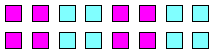Interlocked Phantom Boxes [C4]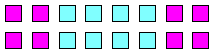Phantom Boxes [C4]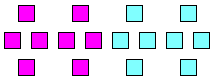Split Phantom Diamonds [C3B]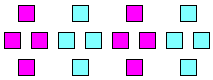Interlocked Phantom Diamonds [C4]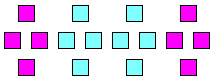Phantom Diamonds [C4]Choreography for Split Phantom Boxes ConceptComments? Questions? Suggestions?

https://www.ceder.net/def/splitphantomboxes.php?level=C3B&language=japan
27-January-2020 10:13:44